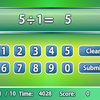# Math search

Solving equations may look like a piece of cake to the math masters, but is it still easy when you need to spot the needle in the haystack? Come and check if you are observant enough in this interesting puzzle game with a mathematical twist! Your goal is to solve the given equations one by one, then locate the answers in a grid which contains numerous numbers. When the game starts, a question will be shown at the lower left corner, and the grid will be located on the right of the screen. After solving the equation, you need to click and drag the corresponding numbers in the grid. The numbers can be read horizontally, vertically or diagonally, and can run in any direction, such as from left to right, right to left, bottom right to upper left, etc. After marking the answer, you can click the Check button at the bottom of the screen to see if it is correct. If your answer is wrong, you need to calculate again in order to proceed. A timer at the top left corner will record the time you hav

## Related software (5)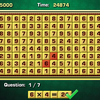## Arithmetic Game

Complete the equations in the interesting arithmetic test!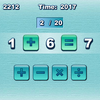## Quick Math

How quickly can you complete the equations with arithmetic symbols?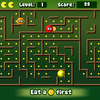## Math Man

Play Ghost Man with a mathematical twist!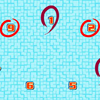## Make 15

Pick 3 numbers that add up to 15.